# How to Create an Excel File Using Visual Basic.Net

0
1271

Today I will teach you about How to Create an Excel File Using Visual Basic.Net. Create an Excel File Using Visual Basic.Net provides support for interoperability between the COM object model of Microsoft Excel and your application. So let’s get started:

• First is open the Visual Basic, Select File on the menu, then click New and create a new project.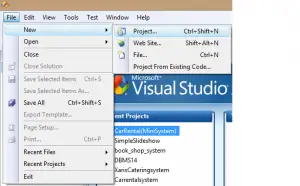• Then a New Project Dialog will appear. You can rename your project, depending on what you like to name it. After that click OK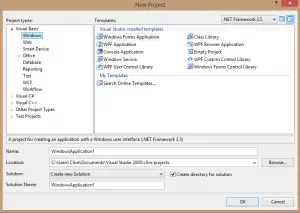• Then design your form like this just like what I’ve shown you below
Add a Button from the toolbox.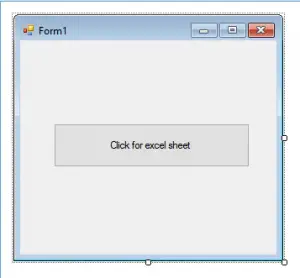• After that, Add a Reference to Microsoft Excel Object Library to your project.

To do this:

*Select add reference from the project menu.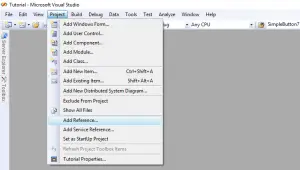*On the COM tab, Locate Microsoft Excel Object Library and then click select.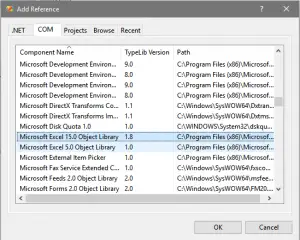*After that, Click OK

• Double click the code window and add this following code to the top of Public Class Form1
[vbnet]

Imports Excel = Microsoft.Office.Interop.Excel

[/vbnet]
• Then, Go back to Design View and Double click the Button and add this following code.
[vbnet]

Private Sub Button1_Click(ByVal sender As System.Object, ByVal e As System.EventArgs) Handles Button1.Click
Dim appXL As Excel.Application
Dim wbXl As Excel.Workbook
Dim shXL As Excel.Worksheet
Dim raXL As Excel.Range
appXL = CreateObject(“Excel.Application”)
appXL.Visible = True
wbXl = appXL.Workbooks.Add
shXL = wbXl.ActiveSheet

shXL.Cells(1, 1).Value = “FIRST NAME”
shXL.Cells(1, 2).Value = “LAST NAME”
shXL.Cells(1, 3).Value = “FULL NAME”
shXL.Cells(1, 4).Value = “SUBJECTS”

With shXL.Range(“A1”, “D1”)
.Font.Bold = True
.VerticalAlignment = Excel.XlVAlign.xlVAlignCenter
End With

Dim pupils(5, 2) As String
pupils(0, 0) = “Markfil”
pupils(0, 1) = “Baldomero”
pupils(1, 0) = “Juvy”
pupils(1, 1) = “Aral”
pupils(2, 0) = “Andrew”
pupils(2, 1) = “Braza”
pupils(3, 0) = “Carlie”
pupils(3, 1) = “Golez”
pupils(4, 0) = “Jesriel”
pupils(4, 1) = “Agita”

shXL.Range(“A2”, “B6”).Value = pupils

raXL = shXL.Range(“C2”, “C6”)
raXL.Formula = “=A2 & “” “” & B2″

With shXL
.Cells(2, 4).Value = “English”
.Cells(3, 4).Value = “Algebra”
.Cells(4, 4).Value = “Physics”
.Cells(5, 4).Value = “Trigonometry”
.Cells(6, 4).Value = “Theology”
End With

raXL = shXL.Range(“A1”, “D1”)
raXL.EntireColumn.AutoFit()

appXL.Visible = True
appXL.UserControl = True

raXL = Nothing
shXL = Nothing
wbXl = Nothing
appXL.Quit()
appXL = Nothing
Exit Sub
Err_Handler:
MsgBox(Err.Description, vbCritical, “Error: ” & Err.Number)
End Sub

[/vbnet]
• Finally, Click F5 to run the program.

## If you have any questions or suggestions about How to Create an Excel File Using Visual Basic.Net please contact me through our contact page.

Our Previous topics: How to Read an Excel File using Visual basic.Net

## Download How to Create an Excel File Using Visual Basic.Net Source code Here

Readers might read also:

Facebook Comments

This site uses Akismet to reduce spam. Learn how your comment data is processed.